### Polynomial Interpolation: Introduction

### Introduction to Polynomial Interpolation

Polynomial interpolation is the procedure of fitting a polynomial of degreeto a set of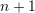data points. For example, if we have two data points, then we can fit a polynomial of degree 1 (i.e., a linear function) between the two points. If we have three data points, then we can fit a polynomial of the second degree (a parabola) that passes through the three points. Figure 1 shows fitting polynomials of degrees 1, 2, 3, and 4, to sets of 2, 3, 4, and 5 data points. The polynomial equation for each curve is shown in the title for each graph.

Polynomial interpolation relies on the fact that for everydata points, there is a unique polynomial of degreethat passes through thesedata points. This fact relies on the Fundamental Theorem of Algebra that states that every degreepolynomial has exactlyroots. So, if two degreepolynomials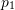and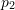agree onpoints, then, their difference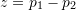is a polynomial of degreebut hasroots which implies that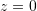.

To find the unique polynomial of degreethat passes throughdata points, we need to solve a linear set ofequations with the coefficients of the polynomial being the unknowns. Let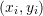be the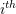data point where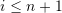. Then, we have: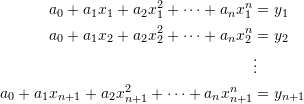Which can be written as follows in matrix form:

(1)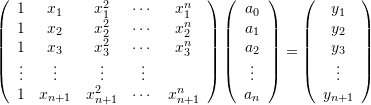The above system can be solved to find the values of the coefficients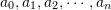which would ensure that the polynomial curve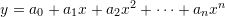passes through thedata points.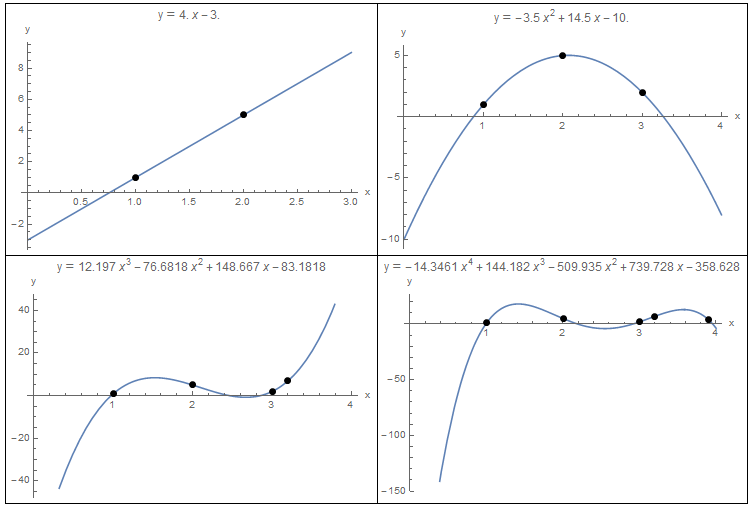Figure 1. Polynomial Interpolation for 2, 3, 4, and 5 data points.

View Mathematica Code
Data = {{1, 1}, {2, 5.}};
y = InterpolatingPolynomial[Data, x];
a = Plot[y, {x, 0, 3}, Epilog -> {PointSize[Large], Point[Data]}, AxesLabel -> {"x", "y"}, ImageSize -> Medium, PlotLabel -> "y" == Expand[y]];

Data = {{1, 1}, {2, 5.}, {3, 2}};
y = InterpolatingPolynomial[Data, x];
b = Plot[y, {x, 0, 4}, Epilog -> {PointSize[Large], Point[Data]},AxesLabel -> {"x", "y"}, ImageSize -> Medium, PlotLabel -> "y" == Expand[y]];

Data = {{1, 1}, {2, 5}, {3, 2}, {3.2, 7}};
y = InterpolatingPolynomial[Data, x];
c = Plot[y, {x, 0, 4}, Epilog -> {PointSize[Large], Point[Data]}, AxesLabel -> {"x", "y"}, ImageSize -> Medium, PlotLabel -> "y" == Expand[y]];

Data = {{1, 1}, {2, 5}, {3, 2}, {3.2, 7}, {3.9, 4}};
y = InterpolatingPolynomial[Data, x];
d = Plot[y, {x, 0, 4}, Epilog -> {PointSize[Large], Point[Data]}, AxesLabel -> {"x", "y"}, ImageSize -> Medium, PlotLabel -> "y" == Expand[y]];
Grid[{{a, b}, {c, d}}, Frame -> All]

View Python Code
import numpy as np
import sympy as sp
import matplotlib.pyplot as plt

x = sp.symbols('x')
x_val = np.arange(0,4,0.01)

Data = [(1, 1), (2, 5.)]
y = sp.interpolate(Data, x)
plt.title(sp.N(y,3))
plt.plot(x_val, [y.subs(x,i) for i in x_val])
plt.scatter([point for point in Data],[point for point in Data], c='k')
plt.grid(); plt.show()

Data = [(1, 1), (2, 5.), (3, 2)]
y = sp.interpolate(Data, x)
plt.title(sp.N(y,3))
plt.plot(x_val, [y.subs(x,i) for i in x_val])
plt.scatter([point for point in Data],[point for point in Data], c='k')
plt.grid(); plt.show()

Data = [(1, 1), (2, 5), (3, 2), (3.2, 7)]
y = sp.interpolate(Data, x)
plt.title(sp.N(y,3))
plt.plot(x_val, [y.subs(x,i) for i in x_val])
plt.scatter([point for point in Data],[point for point in Data], c='k')
plt.grid(); plt.show()

Data = [(1, 1), (2, 5), (3, 2), (3.2, 7), (3.9, 4)]
y = sp.interpolate(Data, x)
plt.title(sp.N(y,3))
plt.plot(x_val, [y.subs(x,i) for i in x_val])
plt.scatter([point for point in Data],[point for point in Data], c='k')
plt.grid(); plt.show()


#### Example

Find an interpolating polynomial that would fit the data points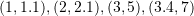.

##### Solution

There are four data points, so, we can fit a third degree polynomial whose coefficients can be found by solving the following system of four linear equations: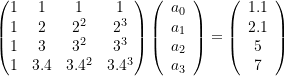Solving the above system yields: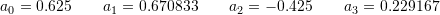Therefore, the interpolating polynomial that would pass through all four data points is: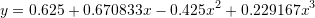The following Mathematica code was used to solve the above equations and produce the plot below:

View Mathematica Code
M = {{1, 1, 1, 1}, {1, 2, 4, 2^3}, {1, 3, 9, 27}, {1, 3.4, 3.4^2, 3.4^3}};
yv = {1.1, 2.1, 5, 7}
a = Inverse[M].yv
y = a[] + a[]*x + a[]*x^2 + a[]*x^3
Plot[y, {x, 0, 4}, Epilog -> {PointSize[Large],  Point[{{1, 1.1}, {2, 2.1}, {3, 5}, {3.4, 7}}]}, AxesLabel -> {"x", "y"}, ImageSize -> Medium, PlotLabel -> "y" == Expand[y]]

View Python Code
import numpy as np
import sympy as sp
import matplotlib.pyplot as plt

M = [[1, 1, 1, 1], [1, 2, 4, 2**3], [1, 3, 9, 27], [1, 3.4, 3.4**2, 3.4**3]]
yv = [1.1, 2.1, 5, 7]
a = np.matmul(np.linalg.inv(M),yv)
x = sp.symbols('x')
y = a + a*x + a*x**2 + a*x**3
x_val = np.arange(0,4,0.01)
plt.plot(x_val, [y.subs(x,i) for i in x_val])
plt.scatter([1, 2, 3, 3.4],[1.1, 2.1, 5, 7], c='k')
plt.title(sp.N(y,5))
plt.grid(); plt.show()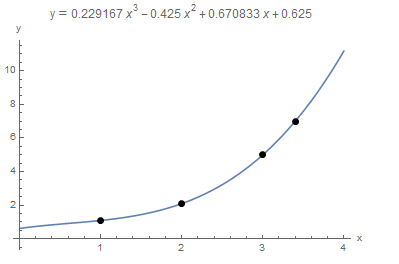MATLAB file:

Mathematica also has a built-in “InterpolatingPolynomial[Data,x]” function that would automatically form and solve the above equation as shown below. Simply provide the data points as a list and Mathematica would produce the interpolating polynomial as a function of “x”.

View Mathematica Code
Data = {{1, 1.1}, {2, 2.1}, {3, 5}, {3.4, 7}};
y = InterpolatingPolynomial[Data, x];
Plot[y, {x, 0, 4}, Epilog -> {PointSize[Large], Point[Data]}, AxesLabel -> {"x", "y"}, ImageSize -> Medium, PlotLabel -> "y" == Expand[y]]

View Python Code
import numpy as np
import sympy as sp
import matplotlib.pyplot as plt

x = sp.symbols('x')
Data = [(1, 1.1), (2, 2.1), (3, 5), (3.4, 7)]
y = sp.interpolate(Data, x)
x_val = np.arange(0,4,0.01)
plt.plot(x_val, [y.subs(x,i) for i in x_val])
plt.scatter([point for point in Data],[point for point in Data], c='k')
plt.title(sp.N(y,3))
plt.grid(); plt.show()


There are various disadvantages to this interpolating polynomials method. The first disadvantage is that as the number of data points gets bigger, the linear systems of equations that need to be solved become ill-conditioned. In other words, the matrix that needs to be inverted would be composed of both small and very large numbers, a situation which is prone to round-off errors. Another major disadvantage is that as the number of data points increases, the order of the interpolating polynomial increases as well. By construction, the polynomial would pass through the data points. However, for large order (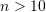), the polynomial would be oscillating wildly between the data points leading to large errors in the interpolated values. The following example illustrates this:

##### Example

Consider the following 11 data points: (-1.00,0.038),(-0.80,0.058),(-0.60,0.100),(-0.4,0.200),(-0.20,0.500),(0.00,1.000),(0.20,0.500),(0.40,0.200),(0.60,0.100),(0.80,0.0580),(1.00,0.038). Using the InterpolatingPolynomial function in Mathematica the 10th order polynomial that would fit through all these points has the form: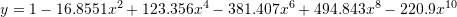From the plot below, the polynomial indeed passes through the 11 points, however, in between the points it oscillates wildly. In fact, for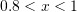the values of the polynomial are much higher than the values at the points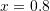and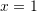which were used for the interpolation: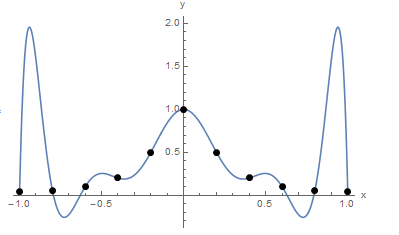It is worth noting that the data points were actually generated using the Runge function: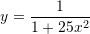When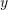is plotted versus the interpolation function, it is clear that the polynomial interpolation function, if used instead ofwould produce erroneous results for values outside of the control points. In fact, Runge used this example in the nineteenth century to show that using higher order polynomials to approximate functions does not always improve accuracy but can have detrimental effects on the values of the function in the intervals between the control points.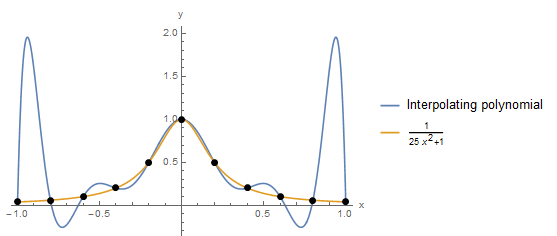View Mathematica Code
Data = {{-1, 0.038}, {-0.8, 0.058}, {-0.60, 0.10}, {-0.4, 0.20}, {-0.2, 0.50}, {0, 1}, {0.2, 0.5}, {0.4, 0.2}, {0.6, 0.1}, {0.8, 0.058}, {1, 0.038}};
y = InterpolatingPolynomial[Data, x]
Chop[Expand[y]]
Plot[y, {x, -1, 1}, Epilog -> {PointSize[Large], Point[Data]}, AxesLabel -> {"x", "y"}, ImageSize -> Medium]
y2 = 1/(1 + 25 x^2)
Plot[{y, y2}, {x, -1, 1}, Epilog -> {PointSize[Large], Point[Data]}, AxesLabel -> {"x", "y"}, ImageSize -> Medium, PlotLegends -> {"Interpolating polynomial", y2}]

View Python Code
import numpy as np
import sympy as sp
import matplotlib.pyplot as plt

x = sp.symbols('x')
x_val = np.arange(-1,1.01,0.01)
Data = [(-1, 0.038), (-0.8, 0.058), (-0.60, 0.10), (-0.4, 0.20), (-0.2, 0.50), (0, 1), (0.2, 0.5), (0.4, 0.2), (0.6, 0.1), (0.8, 0.058), (1, 0.038)]
y = sp.interpolate(Data, x)
plt.plot(x_val, [y.subs(x,i) for i in x_val])
plt.scatter([point for point in Data],[point for point in Data], c='k')
plt.grid(); plt.show()

y2 = 1/(1 + 25*x**2)
plt.plot(x_val, [y.subs(x,i) for i in x_val], label='Interpolating polynomial')
plt.plot(x_val, [y2.subs(x,i) for i in x_val], label=y2)
plt.scatter([point for point in Data],[point for point in Data], c='k')
plt.legend(); plt.grid(); plt.show()

MATLAB file: